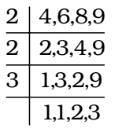## LCM and HCF

#### LCM and HCF

1. The least number which when divided by 16, 18, 20 and 25 leaves 4 as remainder in each case but when divided by 7 leaves no remainder is

1. LCM of 16, 18, 20 and 25 = 3600
∴ Required number = 3600K + 4 which is exactly divisible by 7 for certain value of K.
When K = 5,
Required number = 3600 × 5 + 4

##### Correct Option: D

LCM of 16, 18, 20 and 25 = 3600
∴ Required number = 3600K + 4 which is exactly divisible by 7 for certain value of K.
When K = 5,
Required number = 3600 × 5 + 4 = 18004 , which is exactly divisible by 7.

1. What is the smallest number which leaves remainder 3 when divided by any of the numbers 5, 6 or 8 but leaves no remainder when it is divided by 9 ?

1. We find LCM of 5, 6 and 8
5 = 5
6 = 3 × 2
8 = 23
LCM of 5, 6 and 8 = 23 × 3 × 5 = 8 × 15 = 120
Required number = 120K + 3
when K = 2,

##### Correct Option: D

We find LCM of 5, 6 and 8
5 = 5
6 = 3 × 2
8 = 23
LCM of 5, 6 and 8 = 23 × 3 × 5 = 8 × 15 = 120
Required number = 120K + 3
when K = 2,
∴ Required number = 120 × 2 + 3 = 243
It is completely divisible by 9

1. A number which when divided by 10 leaves a remainder of 9, when divided by 9 leaves a remainder of 8, and when divided by 8 leaves a remainder of 7, is :

1. We know that when a number is divided by a, b or c leaving remainders p, q and r respectively such that the difference between divisor and remainder in each case is same i.e., (a – p) = (b – q) = (c – r) = t (say) then that (least) number must be in the form of (k – t), where k is LCM of a , b and c .
Here, t = Divisor – remainder = 1
t = 10 – 9 = 1, 9 – 8 = 1, 8 – 7 = 1

##### Correct Option: C

We know that when a number is divided by a, b or c leaving remainders p, q and r respectively such that the difference between divisor and remainder in each case is same i.e., (a – p) = (b – q) = (c – r) = t (say) then that (least) number must be in the form of (k – t), where k is LCM of a , b and c .
Here, t = Divisor – remainder = 1
t = 10 – 9 = 1, 9 – 8 = 1, 8 – 7 = 1
∴ Required number = k - t = (L.C.M. of 10, 9, 8) – 1
Hence , Required number = 360 – 1 = 359

1. The smallest number, which when divided by 12 and 16 leaves remainder 5 and 9 respectively, is :

1. As we know that when a number is divided by a, b leaving remainders p, q respectively such that the difference between divisor and remainder in each case is same i.e., (a – p) = (b – q) = t (say) then that (least) number must be in the form of (k – t), where k is LCM of a and b .
Here, t = 12 – 5 = 7, 16 – 9 = 7

##### Correct Option: B

As we know that when a number is divided by a, b leaving remainders p, q respectively such that the difference between divisor and remainder in each case is same i.e., (a – p) = (b – q) = t (say) then that (least) number must be in the form of (k – t), where k is LCM of a and b .
Here, t = 12 – 5 = 7, 16 – 9 = 7
∴ Required number = k - t = (L.C.M. of 12 and 16) – 7
Required number = 48 – 7 = 41

1. The least number which when divided by 4, 6, 8 and 9 leave zero remainder in each case and when divided by 13 leaves a remainder of 7 is :

1. LCM of 4, 6, 8, 9∴ LCM = 2 × 2 × 3 × 2 × 3 = 72

##### Correct Option: B

LCM of 4, 6, 8, 9∴ LCM = 2 × 2 × 3 × 2 × 3 = 72
∴ Required number = 72, because it is exactly divisible by 4, 6, 8 and 9 and it leaves remainder 7 when divided by 13.